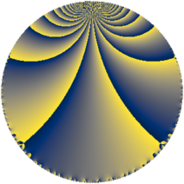# Properties

 Label 96.4.nLevel $96$ Weight $4$ Character orbit 96.n Rep. character $\chi_{96}(13,\cdot)$ Character field $\Q(\zeta_{8})$ Dimension $96$ Newform subspaces $1$ Sturm bound $64$ Trace bound $0$

# Related objects

## Defining parameters

 Level: $$N$$ $$=$$ $$96 = 2^{5} \cdot 3$$ Weight: $$k$$ $$=$$ $$4$$ Character orbit: $$[\chi]$$ $$=$$ 96.n (of order $$8$$ and degree $$4$$) Character conductor: $$\operatorname{cond}(\chi)$$ $$=$$ $$32$$ Character field: $$\Q(\zeta_{8})$$ Newform subspaces: $$1$$ Sturm bound: $$64$$ Trace bound: $$0$$

## Dimensions

The following table gives the dimensions of various subspaces of $$M_{4}(96, [\chi])$$.

Total New Old
Modular forms 200 96 104
Cusp forms 184 96 88
Eisenstein series 16 0 16

## Trace form

 $$96q + O(q^{10})$$ $$96q - 120q^{10} + 48q^{12} + 416q^{14} + 120q^{16} + 72q^{18} - 160q^{20} + 40q^{22} - 656q^{23} - 456q^{24} - 40q^{26} - 760q^{28} + 1488q^{31} - 1240q^{32} - 1000q^{34} + 912q^{35} + 440q^{38} + 1352q^{40} - 1616q^{43} + 1000q^{44} + 1440q^{46} + 2856q^{50} + 1488q^{51} + 2520q^{52} - 1504q^{53} + 216q^{54} + 288q^{55} - 392q^{56} - 2016q^{58} - 2752q^{59} - 2448q^{60} + 1824q^{61} - 2928q^{62} - 1008q^{63} - 6888q^{64} - 2736q^{66} - 816q^{67} - 5032q^{68} + 1056q^{69} - 1800q^{70} - 448q^{71} + 6944q^{74} + 2208q^{75} + 5032q^{76} - 3808q^{77} + 3528q^{78} + 11336q^{80} + 9320q^{82} + 2368q^{86} - 944q^{88} + 3600q^{91} - 7696q^{92} - 16480q^{94} - 5160q^{96} - 10288q^{98} + O(q^{100})$$

## Decomposition of $$S_{4}^{\mathrm{new}}(96, [\chi])$$ into newform subspaces

Label Dim. $$A$$ Field CM Traces $q$-expansion
$$a_2$$ $$a_3$$ $$a_5$$ $$a_7$$
96.4.n.a $$96$$ $$5.664$$ None $$0$$ $$0$$ $$0$$ $$0$$

## Decomposition of $$S_{4}^{\mathrm{old}}(96, [\chi])$$ into lower level spaces

$$S_{4}^{\mathrm{old}}(96, [\chi]) \cong$$ $$S_{4}^{\mathrm{new}}(32, [\chi])$$$$^{\oplus 2}$$